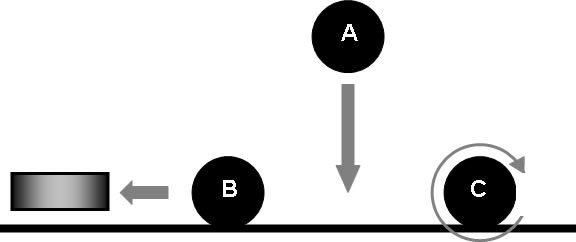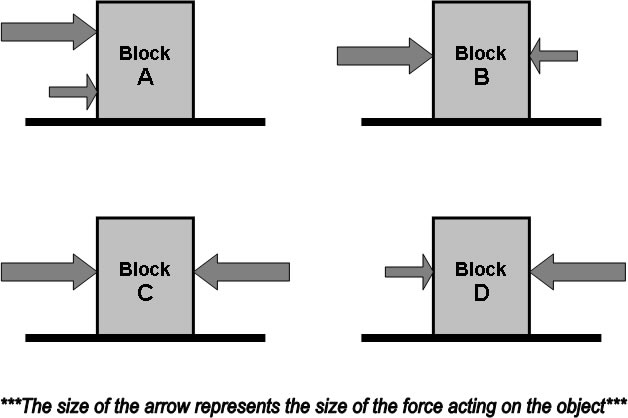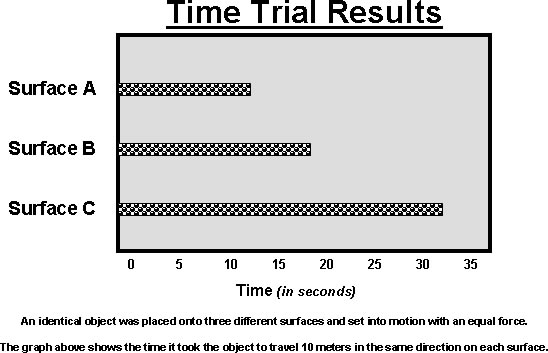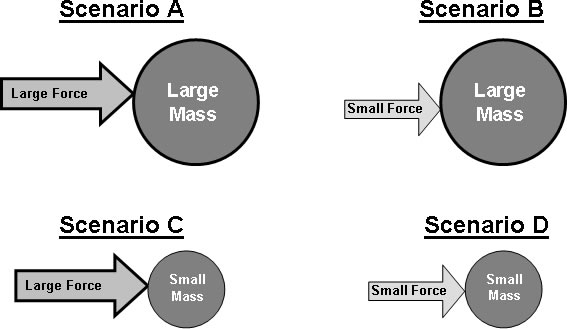Forces and Motion Test
starstarstarstarstarstarstarstarstarstar
by Anna Scales
| 15 Questions1
1
"Ball A" was dropped from a higher elevation. Which of the following forces is responsible for it falling downward?
gravity
electricity
magnetism
friction2
1
"Ball B" is moving towards the rectangular block. Which feature of the block can explain why this is happening?
the block is blowing air towards "Ball B"
the block is a magnet (and "Ball B" is made of a magnetic material
the block is heavier than "Ball B"
the block is located on the highest part of the surface3
1
"Ball C" is rolling across the surface, but it eventually comes to a stop. Which force is most likely responsible for this?
electricity
gravity
friction
magnetism4
1
The graph above shows a race between Swimmer 1 and Swimmer 2. In the end, Swimmer 2 was the winner. Which of the following could explain this result?
There were other competitors in the race.
Swimmer 1 was heading in the wrong direction.
The race was for only 50 meters.
Swimmer 2 was moving faster than Swimmer 1.5
1
Based on the graph above, it can be determined that:
Swimmer 1 is faster than Swimmer 2
Swimmer 1 and Swimmer 2 were moving in different direction
Swimmer 2 is faster than Swimmer 1
Swimmer 1 and Swimmer 2 were moving in the same direction6
1
Based on the above graph, which of the following predictions could be made?
Swimmer 2 is not able to swim 100 meters.
Swimmer 2 would be better in long-distance races.
Swimmer 1 is faster than the average swimmer.
It would take twice as long for Swimmer 2 to swim 100 meters than Swimmer 1.7
1
Which of the blocks shown above would not move when exposed to the forces above?
Block C
Block D
Block A
Block B8
1
If all of the above blocks are the same mass, which of the following would slide across the surface the fastest as a result of the forces acting on it?
Block A
Block D
Block B
Block C9
1
If Block B and Block D are the same mass, which of the following describes how they would move when exposed to the forces above?

Both blocks would move in the same direction and at the same speed.
Both blocks would move in the same direction, but at different speeds.
Both blocks would stay still.
Both blocks would move at the same speed, but in different directions.10
1
A graph like the one above might be useful when trying to decide what?

the proper width to build a road and keep traffic flowing smoothly
the best material to use when constructing a new road (gravel, asphalt, concrete, etc.)
the fastest way to drive from one part of town to another
the safest height to build a bridge crossing over a river11
1
If the graphs above are describing the motion of a sled, which of the following might explain why Surface A was the fastest?
it was the only surface that sloped upward
it was the roughest and dryest surface
it was the only surface that was lubricated and slippery
it had more surface area touching the object than the other surfaces12
1
An identical object was placed onto the three different surfaces and set into motion with an identical force. The bars show how fast it took the object to travel 10 meters. Based on the graph, which surface had the greatest friction?
Friction is the same on every surface
Surface B
Surface A
Surface C13
1
In Scenario A, a ball is being pushed along by a large force. If the force were suddenly decreased (like in Scenario B), what would happen to the motion of the ball?
it would remain the same
it would change direction
it would slow down
it would speed up14
1
The ball in Scenario D is moving at the same speed as the ball in Scenario A. This is because:
the ball in Scenario D must have unseen forces acting on it.
the ball in Scenario D has a smaller diameter, but it is the same weight as the ball in Scenario A.
the surface in Scenario D is lubricated.
the relationship of force to mass are the same in both scenarios.15
1
If the forces are the same in Scenario A and Scenario C, which ball would move the fastest?
the ball in Scenario C
there is no way to determine which ball would move the fastest
both balls would move at the same speed
the ball in Scenario A